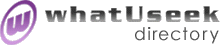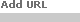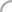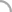SearchThe Directory The Webfor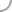AlgebraistsNew! Submit a site

whatUseek Collection Sites (submit a site ):

Give your site great placement in this category in as little as two business days!

whatUseek Directory Site Listings:

Cramer - Gabriel Cramer (1704-1752) - Best known for his work on determinants, made contributions to the study of algebraic curves.

Dedekind, Richard (1831-1916) - study of CONTINUITY and definition of the real numbers in terms of Dedekind "cuts", the nature of number and mathematical induction, definition of finite and infinite sets; algebraic number fields, concept of RINGS.

Galois, Évariste (1811-1832) - Galois theory, a branch of mathematics dealing with the general solution of equations, group theory, method of determining when a general equation could be solved by radicals, solved many long-standing unanswered questions.

Niels Henrik Abel, Norwegian mathematician - Biography of the mathematician Niels Henrik Abel and a description of his work, such as with the quintic equation.

Pell, John (1611-1685) - Worked on algebra and number theory, gave a table of factors of all integers up to 100000 in 1668. Pell's equation is y^2 = ax^2 + 1, where a is a non-square integer.

Schmidt, Erhard (1876-1959) - Main research was functional analysis, doctorate was obtained under Hilbert's supervision, main interest was in integral equations and Hilbert space, best remembered for the Gram-Schmidt orthogonalisation process.

Zermelo - Ernst Friedrich Ferdinand Zermelo (1871-1953) - Zermelo in 1908 was the first to attempt an axiomatisation of set theory

al-Khwarizmi - Abu Ja'far al-Khwarizmi (780-850) - Arab mathematician, lived when spread of ideas was very slow, wrote Hisab al-jabr wal-muqabala, concepts of the Middle East. The "al-jabr" gave us the word algebra." introduced the decimal system, rules for solving linear and quadratic equations, the word "algorithm" comes from his name.Help build the largest human-edited directory on the web.About   Help   Content Filter   Terms   Privacy Policy© 2018 whatUseek